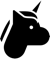I'm looking for a way to get the difference between 2 input CVs. For example, CV1 = 1V, CV2 = 1.5V, Out = .5V
Ideally, it would work with negative output as well, CV1= 1V, CV2 = -2V, Out = -3V
I think what I'm looking for is a comparator but all the modules I find seem to have outputs that are either low or high when CV2 is compared to CV1. I'm not sure what the name is of what I'm looking for, or if there is a way to do it with modules such as Math?Hi mate,
I guess the word you are looking for is subtraction. Maths does that with ease. Maybe you want to look at this fine piece of work that someone put together...
Maths illustrated supplement (Page 15 should be about right)

In my opinion it's a must read for maths users. On the other hand there is the Addac 216 that has the subtitle sum&difference. If you fancy a dedicated module for that purpose.

Have funHi Artvark,

The Shakmat Modular - SumDif module, just 2 HP, can do this as well. Dual adder and/or subtraction.

Kind regards, Garfield.This is what you're looking for: https://www.modulargrid.net/e/shakmat-modular-sumdif That, or something along the same lines. The Shakmat module here can do addition and subtraction of CV values, but any adder will also work because if you send an adder a negative voltage, it'll treat that as a subtractive operation.Hi Lugia,

Ha, ha, at almost the exact same time we had the exact same thoughts ;-)

Kind regards, Garfield.Thanks for the input. I don't think it can be done with Maths, it does addition and subtraction but not the difference.
That Shakmat SumDif seems to be exactly what I'm looking for. I will take a look at adders too.

Thx again :-)You are looking for an analog logic module! Mystic circuits Ana is my top recommendation.
https://youtu.be/Q49hvFyL8You are looking for an analog logic module! Mystic circuits Ana is my top recommendation.
https://youtu.be/Q49hvFyL8
-- Miszczyk

Save that that's not exactly a "logic" module...it has some very useful functions, however, including multiplication of CV levels. But "logic" for the most part refers to the actual Boolean gates themselves, while this is more akin to an adder/4-quadrant multiplier/discriminator. Definitely does more than the SumDif, but the question then becomes whether or not the OP can fit that into their build by freeing up 4 hp somewhere.

Maybe MG needs another category for "CV Arithmetics"...? It's not exactly "modulation", because these recalculate the CV level instead of imposing a modulation curve on them. And it's not "logic" since there's no status-dependent digital on/off states. Nor is this a "comparator" as such...hm...The Mystic Circuits Ana should do the job, but I want to see if I can solve it with my existing modules. I think what I can do is use Maths subtraction, and then invert negative results. So:
CV1 = 2V, CV2= 1V, Subtraction = 1V
CV1 = 3V, CV2 = 5V, Subtraction = -2V, invert that to get my 2V

FYI, I want to use this with a Theremin. I don't play well enough to play in tune with it but I do not want to just quantize the output either, may as well play keyboard then. So the idea is split the CV out, quantize 1 side of the split and compare that to the unquantized signal. The difference is then used to drive something else. Could be a modulation depth of sorts: hit exactly the right CV on the Theremin, you get the 'pure' note/tone. The more you drift away from the correct pitch the greater the voltage difference, the greater the modulation depth. Until you are far enough away from the quantized pitch to hit another note and then the unquantized voltage is compared to that again.
This will lead to some odd results as most scales will not have equidistant 'gaps' in CVs but the effect could be nice. And hide that I cannot play Theremin to save my life.

I'm a bit surprised this 'get the difference' function is not more common, I think you can use it for various interesting purposes.A summer (or precision adder) and an inverter will also do the trick.
A - B = A + (-B)Maybe i'm totally wrong here, but what is exactly the "difference" between difference and the result of a subtraction? I'm pretty sure the result of a subtraction is called difference.

To this point...
CV1 = 3V, CV2 = 5V, Subtraction = -2V, invert that to get my 2V
I think i would just switch the two voltages, if that is possible in your use case.
CV1 = 5V, CV2 = 3V, Subtraction = 2V

I mean it's always good to know about alternatives, but Maths does all of this without a problem. I wouldn't even bother to use an inverter. A Full-Wave Rectifier makes more sense imho. MI Kinks has one.